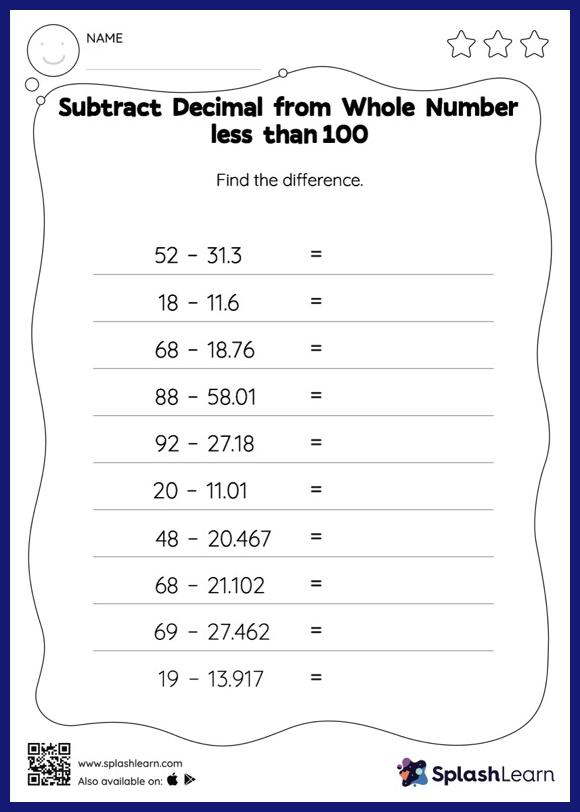# Subtract Decimal from Whole Number less than 100: Horizontal Subtraction Worksheet

Home > Subtract Decimal from Whole Number less than 100: Horizontal SubtractionCalling all young math geniuses! This worksheet challenges 5th graders to work with subtract decimals and helps them sharpen their decimals skills.